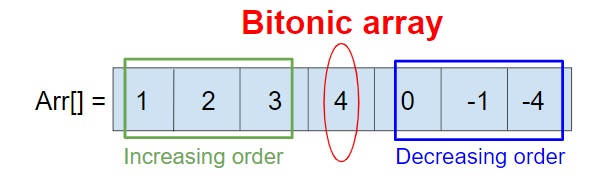# Program to check bitnoicity of an array in C++

Given an array arr[N] of N integers, the task is to check whether the given array is bitonic or not. If the given array is bitonic then print “Yes its a bitonic array”, else print “No its not a bitonic array”.

A Bitonic array is when the array is in strictly increasing order first and then in strictly decreasing order.

Like this array arr[] = {1, 2, 3, 4, 2, -1, -5} is a bitonic array, because till 4 it is in strictly increasing order and after 4 it is in strictly decreasing order.Input

arr[] = {1, 3, 5, 4, 2, 0}

Output

Yes its a bitonic array

Explanation

1< 3 < 5 > 4 > 2 >0, so yes it is a bitonic array.

Input

arr[] = {1, 2, 3, 4, 5, 0, -1, -2, 6, -4}

Output

No its not a bitonic array

## Approach used below is as follows to solve the problem

• Iterate every element of an array and check that the previous element is smaller than the current element.

• When the previous element is not smaller than the current element break.

• Check if the previous element is larger than the current, else return false and exit.

• If reached the end of an array return true.

## Algorithm

Start
Step 1→ Declare array to check for bitonicity of an array
int check(int arr[], int size)
declare int i, j
Loop For i = 1 and i <size and i++
IF (arr[i] > arr[i - 1])
Continue
End
IF (arr[i] <= arr[i - 1])
break
End
IF(i == size - 1)
return 1
End
Loop For (j = i + 1 and j < size and j++
IF (arr[j] < arr[j - 1])
Continue
End
IF (arr[j] <= arr[j - 1])
break
End
End
Set i = j
IF (i != size)
return 0
End
return 1
Step 2→ In main()
Declare int arr[] = { -3, 9, 11, 20, 17, 5, 1 }
Declare int size = sizeof(arr) / sizeof(arr)
Do (check(arr, size) == 1) ? cout << "Yes its a bitonic array" : cout << "no its not a bitonic array"
Stop

## Example

Live Demo

#include <bits/stdc++.h>
using namespace std;
//function to check bitonic or not
int check(int arr[], int size){
int i, j;
for (i = 1; i < size; i++){
if (arr[i] > arr[i - 1])
continue;
if (arr[i] <= arr[i - 1])
break;
}
if (i == size - 1)
return 1;
for (j = i + 1; j < size; j++){
if (arr[j] < arr[j - 1])
continue;
if (arr[j] >= arr[j - 1])
break;
}
i = j;
if (i != size)
return 0;
return 1;
}
int main(){
int arr[] = { -3, 9, 11, 20, 17, 5, 1 };
int size = sizeof(arr) / sizeof(arr);
(check(arr, size) == 1) ? cout << "Yes its a bitonic array" : cout << "no its not a bitonic array";
return 0;
}

## Output

If run the above code it will generate the following output −

Yes its a bitonic array